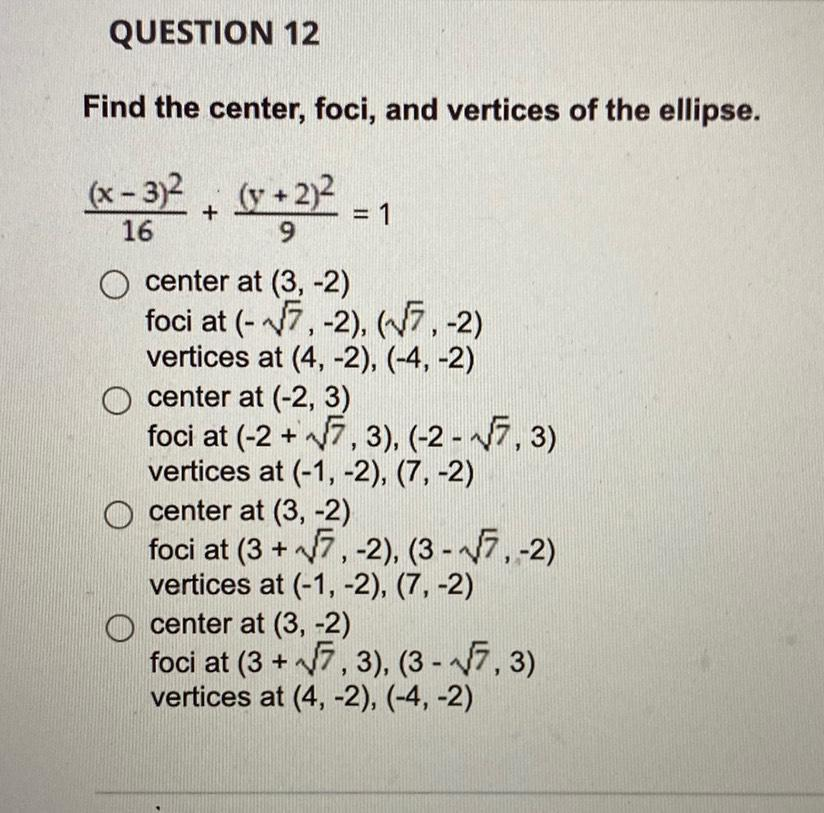Question:

# QUESTION 12 Find the center, foci, and vertices of the ellipse. (x - 3)2, (y + 2)2 + = 1 16 9 O center at (3,-2) foci at (-7, -2QUESTION 12 Find the center, foci, and vertices of the ellipse. (x - 3)2, (y + 2)2 + = 1 16 9 O center at (3,-2) foci at (-7, -2), (-7, -2) vertices at (4, -2), (-4,-2) O center at (-2, 3) foci at (-2+7,3), (-2 - - 1,3) vertices at (-1, -2), (7, -2) O center at (3,-2) foci at (3 + 17, -2), (3 - 7,-2) vertices at (-1,-2), (7,-2) O center at (3,-2) foci at (3 + 17,3), (3 - 17.3) vertices at (4, -2), (-4,-2)